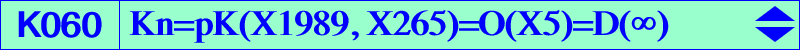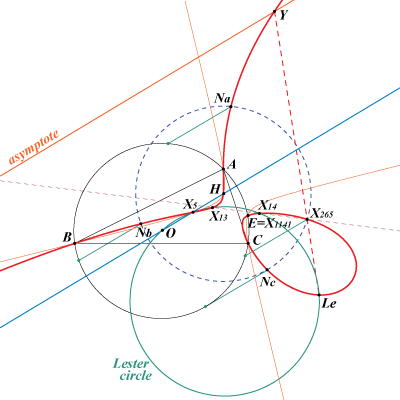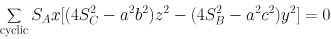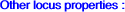X(4), X(5), X(13), X(14), X(30), X(79), X(80), X(265), X(621), X(622), X(1117), X(1141), X(5627), X(11584), X(14372), X(14373) extraversions of X(79) and X(80) Ix-anticevian points : see table 23 points Na, Nb, Nc mentioned in Central CubicsRemember that the Neuberg cubic is the locus of point M such that the triangles ABC and MaMbMc are in perspective, where Ma, Mb, Mc are the reflections of M about the sidelines of ABC. This means that the lines AMa, BMb, CMc are concurrent at point N and then the locus of N is the cubic we call Kn. MaMbMc is called 2-pedal triangle in Pinkernell's paper and Kn is the 2-cevian cubic associated to the 2–pedal cubic (Neuberg cubic) and the –2–pedal cubic (Napoleon cubic). See the related quartic Q161 where a list of pairs {M, N} is given. Kn is the circular pivotal cubic with pivot X(265) = isogonal of inverse of H and pole a point named Po = X(1989) = isogonal of X(323). X(323) is the reflection of X(23) about X(110), where X(23) is the inverse of G and X(110) is the focus of the Kiepert parabola. Its singular focus (not on the curve) is X(3448), the reflection of X(110) about X(125) or the reflection of X(399) about X(5). Its orthic line is the Euler line. Kn is tangent at A, B, C to the altitudes of triangle ABC. Kn is tangent to the parallels to the Euler line at the in/excenters (those lines are also tangent to the Neuberg cubic), the contacts being X(79) and its extraversions. The fourth real intersection E with the circumcircle is the second intersection of the line X(5)X(110) with the circumcircle and also the sixth intersection with the rectangular hyperbola through A, B, C, H, X(5). This point is now called X(1141) in ETC. Its real asymptote is parallel to the Euler line at X(399) or X(323). Kn intersects its asymptote at Y = X(14451) on the line through X(265) and the antigonal of X(399), this latter point is X(1117) (labelled Le on the figure) lying on the Lester circle and on Kn. Kn is also the isogonal transform of K073, the inversive image of the Neuberg cubic in the circumcircle. In other words, Kn is the antigonal transform of the Neuberg cubic. Hence the antigonal of each point on the Neuberg cubic is a point on Kn and vice versa. Kn is a member of the class CL024 of cubics. It is anharmonically equivalent to the Neuberg cubic. See Table 20. The isotomic transform of Kn is K636, its anticomplement is K753 and its complement is K900. K060 and Q155 are both globally invariant under the inversion with pole X(79) which swaps X(80) and X(265).locus of point Q center of perspective of ABC and AmBmCm where M is on the Neuberg cubic and Am is the isogonal conjugate of A with respect to triangle MBC (Bm and Cm defined similarly). Pa, Pb, Pc are the reflections of point P in the sidelines of triangle ABC. Oa, Ob, Oc are the circumcenters of triangles PaBC, PbCA, PcAB. The triangles ABC and OaObOc are perspective if and only if P lies on Kn (together with the circumcircle and the line at infinity). The locus of the perspector is the Napoleon cubic K005. Pa, Pb, Pc are the reflections of point P in the sidelines of triangle ABC and A'B'C' is the cevian triangle of P. The triangles are perspective if and only if P lies on Kn (together with the line at infinity). Kn is O(X5) orthopivotal cubic with orthopivot X(5) : Kn is the locus of P such that X(5), P and the orthocorrespondent of P are collinear. See the FG paper "Orthocorrespondence and orthopivotal cubics" in the Downloads page and Orthopivotal cubics in the glossary. Equivalently, it is the locus of the perspectors of the equilateral triangles centered at X(5) which are perspective with ABC. Locus of pivots of circular pKs which pass through the Fermat points X(13), X(14). The pole lies on K095 and the isopivot lies on K001. Locus of pivots of circular pKs whose orthic line passes through the centroid of ABC. The locus of the poles is K095. See CL035. Let ABC be a triangle and P a point. The reflection of the line AP about BC intersects AB, AC in Ac, Ab respectively. Denote by (Ha) the hyperbola passing through the midpoints of BC, AAb, AAc and whose asymptotes are parallel to the sidelines AB, AC. Define (Hb) and (Hc) similarly. The centers of (Ha), (Hb), (Hc) are collinear if and only if P lies on K060. (Angel Montesdeoca, 2014-06-06) Locus of isopivots of pK60+. The locus of the poles is K095 and the locus of the pivots is K001. See also K001, property 3. See also K005, property 10.